SurfaceColor

The usage message for SurfaceColor is shown below.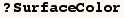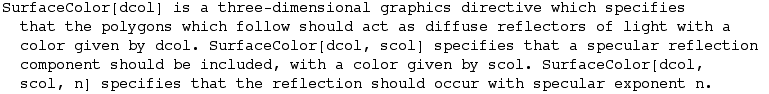Further explanation can be found under SurfaceColor  in the HelpBrowser and section  2.9.12 of The Mathematica Book.  The Mathematica lighting model accounts for difuse light reflection, and specular light reflection. The SurfaceColor directive is used to specify the type of reflection for  Graphics3D primitives.

Difuse Reflection

The default lighting model in Mathematica uses only difuse reflection. With difuse reflection light is equally dispursed in all directions such as the light reflected from cloth or flat paint. When SurfaceColor is used with one argument only difuse reflection is used.

An example is given below to demonstrate the effect of difuse lighting, but first the next cell should be evaluated to make the graphics of a surface with a slight curve shown  at four slightly different views.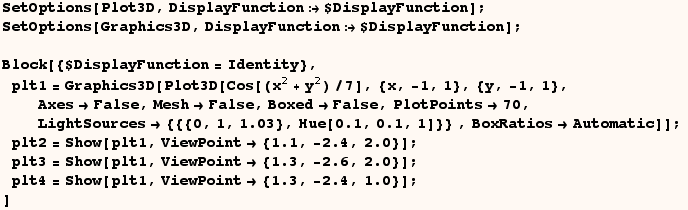The next cell shows the four views above with only difuse reflection.  Notice essentially the same color is reflected from different areas on the surface.  However, the right most view shows the surface with a lighter shade as a result of the way the surface is oriented relative to the light source.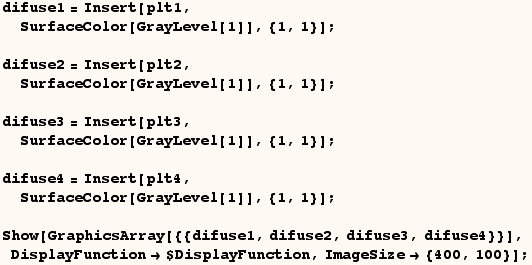Computing the color of difuse reflected light

When the SurfaceColor directive has one argument only difuse reflection is used.  If that argument is GrayLevel[g] then the red, green, and blue components of light reflected from each light source is the red, green, blue components of the incident light times (g Cos[α]) where α is the angle between (the vector normal to the surface of a polygon) and (the direction of the incident light beam).  If the only argument in SurfaceColor is RGBColor[r,g,b] then the red, green, blue compenets of reflected light for each light source are proportional to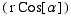,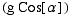,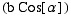respectively.  It seems the only color directives that can be used in SurfaceColor are GrayLevel and RGBColor.

Sepcular Reflection

Mathematica can also model specular reflection where reflected light is concentrated in one direction. A practical example of something that reflects light in this manner is the crome plated bumper on a car.  When SurfaceColor has two or three arguments the first argument specifies the amount of difuse reflection and the second argument specifies the amount of specular reflection.

The next cell shows the same graphics that were used above with specular reflection but no difuse reflection. In the view on the left light is only reflected from a spot in the center of the graphic. When a different ViewPoint is used light is reflected from a different spot on the surface.  In the right most view the surface is oriented such that no specular reflection is visible.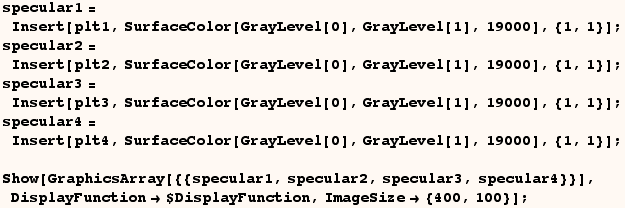Computing the color of Specular reflected light

The red, green, and blue components of difuse reflection and specular reflection from each light source are combined to determine the color used for rendering each polygon. The second argument in SurfaceColor specifies the type of specular reflection. Suppose the second argument of SurfaceColor is GrayLevel[g] and the third argument is n (a positive Real, Integer, or Rational).  In that case the red, green and blue components of specular reflected light are the red, green, blue components of incident light times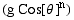where θ is the angle between (the direction the polygon is veiwed from) and (the direction in which light would reflect if the surface was a perfect mirror).  However, there is no specular reflection when θ is 90° or greater. If the second argument in SurfaceColor is RGBColor[r,g,b] then the red, green, and blue compenets of specular reflected light for each light source are proportional to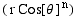,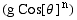,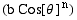.  Here too no light is reflected for θ>90°. When SurfaceColor has only two arguments the default value n=1 is used for the third argument.  It seems the only color directives that can be used in SurfaceColor are GrayLevel and RGBColor.

Examples using a Torus

In the cells that follow a torus is shown using different SurfaceColor directives.  First the next cell should be evaluated so the graphic of a torus can be made.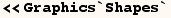The next cell makes a torus and displays it with the default lighting effects.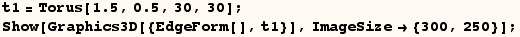In the next cell the SurfaceColor specified has one argument which causes the Torus to come out like the one from the previous cell except a little gray.  The color used for each polygon is determined by multiplying the each component (red, green, blue) of the incident light from each light source by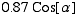where α is the angle between (the vector normal to the surface of a polygon) and (the direction of the incident light beam).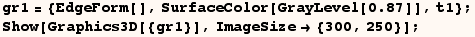In the next cell SurfaceColor has one argument (an RGBColor).   Here again only difuse reflection is used, and the Torus comes out green. In this case the color used for each polygon is determined by multiplying each component (red, green, blue) of the incident light by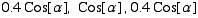respectively.  This is done for each light source and the reflected light form each source is combined to give the total effect.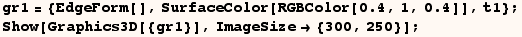In the next cell difuse reflection is used to make the Torus look red.  Here the calculation of the color for each polygon is similar to that used in the last example.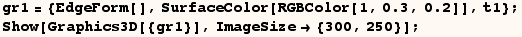When SurfaceColor is given two arguments the first argument indicates the color and amount of difuse reflection to use, and the second argument indicates the color and amount of specular reflection to use. In the next cell the first argument of SurfaceColor the black (GrayLevel).  This causes the use of no difuse lighting, and all reflection of light is governed by the second argument which specifies the specular reflection. The second argument in SurfaceColor causes the intensity of the red, green, blue components of light reflected from each polygon to be proportional to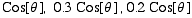respectively.  As discussed above θ is the angle between (the direction the polygon is veiwed from) and (the direction in which light would reflect if the surface was a perfect mirror), except if θ is 90° or greater there is no specular reflection.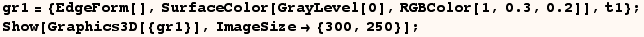The next cell is the same as the last cell except for the third argument in SpecularColor. In this case the third argument in SurfaceColor is 3, so the intensity of the red, green, blue components of light reflected from each polygon is proportional to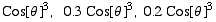respectively.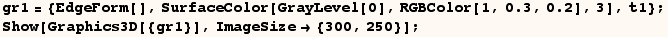The next cell combines the effects of some of the previous examples.  In this case we have green difuse reflection and red specular reflection.  Where both types of reflection are present green and red combine to make yellow.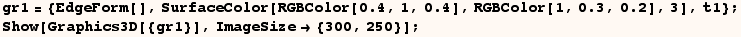The next two cells use a higher and lower value for the third argument in SurfaceColor.  This gives a larger or smaller region with yellow reflection.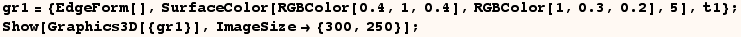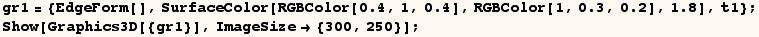The next cell shows three Torus in the same graphic using the default SurfaceColor.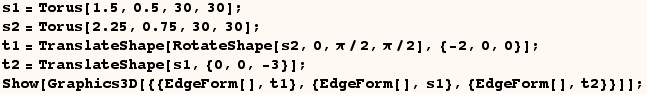In the next cell we can combine objects using different specifications for SurfaceColor.  The next example shows the same three Torus as the last example, but this time they each have a different SurfaceColor.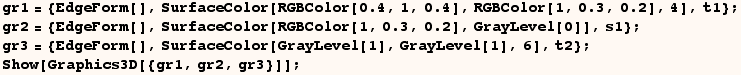SurfaceColor for SurfaceGraphics

If we want to use SurfaceColor when showing Graphics3D as in
Show[Graphics3D[{SurfaceColor[___], s }]]
Then we should provide (s) which is a list of polygons.  Actually SurfaceColor can affect the lighting of Cuboids as well, but we won't look into that right now.  Suppose you want to use SurfaceColor with the graphics produced by Plot3D in the next cell.

plt1=Plot3D[Sin[x y],{x,0.5,2.5},{y,0.5,2.5}];

The expression returned by Plot3D has the head SurfaceGraphics.  By using Graphics3D[plt1] we can convert the SurfaceGraphics to Graphics3D.  We can then insert a SurfaceColor directive at the appropriate position if we don't want to use the default surface.

gr4=Insert[Graphics3D[plt1],SurfaceColor[RGBColor[0,0.3,0.6],RGBColor[0,0.7,0.3],2],{1,1}];
Show[gr4];

We can even combine the graphics from Plot with a Torus that uses a specified SurfaceColor as in the next cell !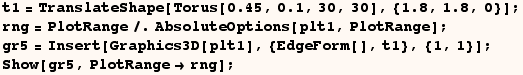Created by Mathematica  (May 16, 2004)

Back to Ted’s Tricks index page